# ggplot2 - Introduction to geoms

### Introduction

This is the third post in the series Elegant Data Visualization with ggplot2. In the previous post, we learnt how to create plots using the `qplot()` function. In this post, we will create some of the most routinely used plots to explore data using the `geom_*` functions.

### Libraries, Code & Data

We will use the following libraries in this post:

All the data sets used in this post can be found here and code can be downloaded from here.

### Data

``````ecom <- readr::read_csv('https://raw.githubusercontent.com/rsquaredacademy/datasets/master/web.csv')
ecom``````
``````## # A tibble: 1,000 x 11
##       id referrer device bouncers n_visit n_pages duration country purchase
##    <dbl> <chr>    <chr>  <lgl>      <dbl>   <dbl>    <dbl> <chr>   <lgl>
##  1     1 google   laptop TRUE          10       1      693 Czech ~ FALSE
##  2     2 yahoo    tablet TRUE           9       1      459 Yemen   FALSE
##  3     3 direct   laptop TRUE           0       1      996 Brazil  FALSE
##  4     4 bing     tablet FALSE          3      18      468 China   TRUE
##  5     5 yahoo    mobile TRUE           9       1      955 Poland  FALSE
##  6     6 yahoo    laptop FALSE          5       5      135 South ~ FALSE
##  7     7 yahoo    mobile TRUE          10       1       75 Bangla~ FALSE
##  8     8 direct   mobile TRUE          10       1      908 Indone~ FALSE
##  9     9 bing     mobile FALSE          3      19      209 Nether~ FALSE
## 10    10 google   mobile TRUE           6       1      208 Czech ~ FALSE
## # ... with 990 more rows, and 2 more variables: order_items <dbl>,
## #   order_value <dbl>``````

#### Data Dictionary

• id: row id
• referrer: referrer website/search engine
• os: operating system
• browser: browser
• device: device used to visit the website
• n_pages: number of pages visited
• duration: time spent on the website (in seconds)
• repeat: frequency of visits
• country: country of origin
• purchase: whether visitor purchased
• order_value: order value of visitor (in dollars)

### Scatter Plot

A scatter plot displays the relationship between two continuous variables. In ggplot2, we can build a scatter plot using `geom_point()`. Scatterplots can show you visually

• the strength of the relationship between the variables
• the direction of the relationship between the variables
• and whether outliers exist

#### Point

The variables representing the X and Y axis can be specified either in `ggplot()` or in `geom_point()`. We will learn to modify the appearance of the points in a different post.

``````ggplot(ecom, aes(x = n_pages, y = duration)) +
geom_point()``````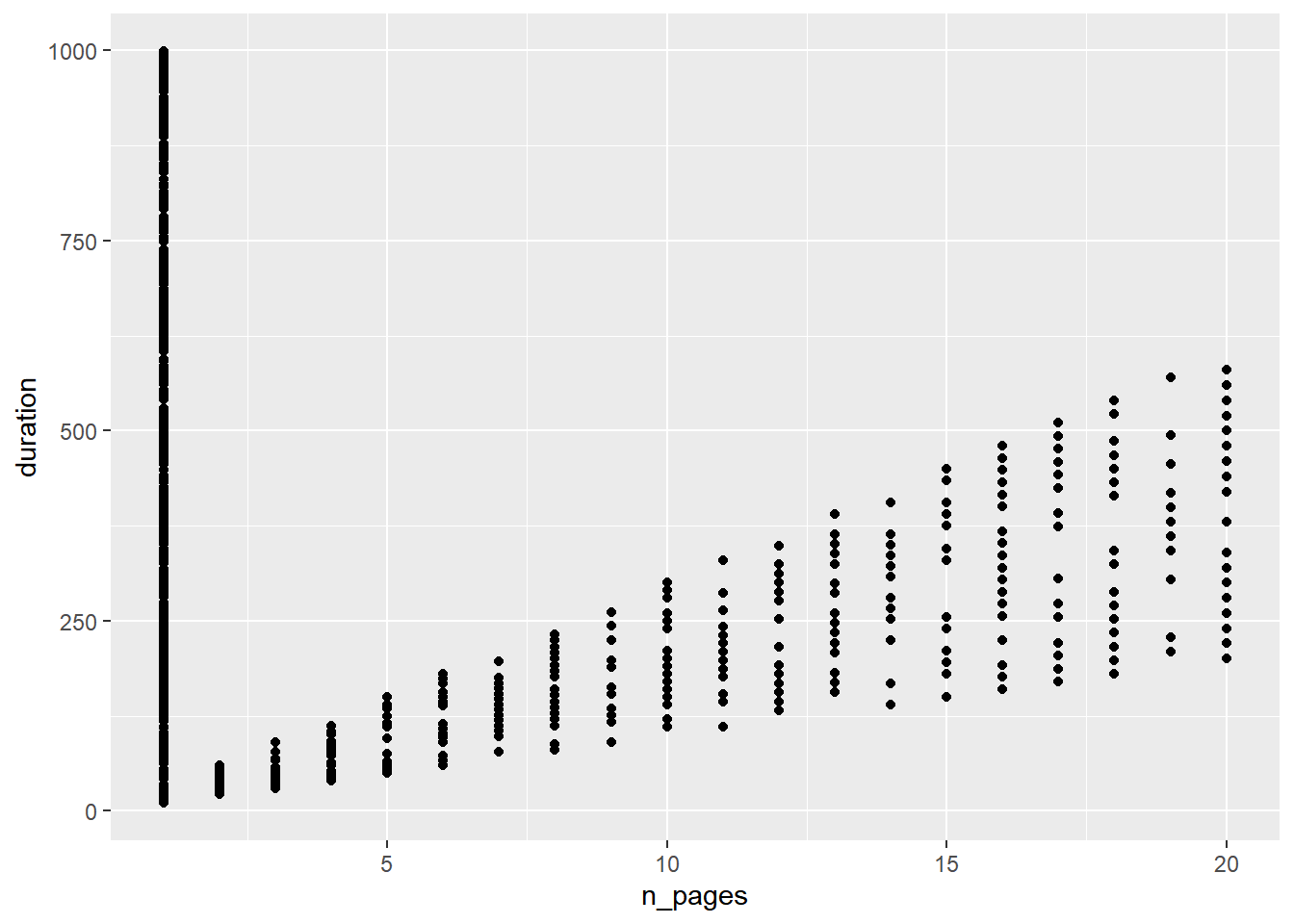#### Regression Line

A regression line can be fit using either:

• `geom_abline()`
• `geom_smooth()`

#### Regression Line

If you are using `geom_abline()`, you need to specify the intercept and slope as shown in the below example:

``````ggplot(mtcars, aes(x = wt, y = mpg)) +
geom_point() +
geom_abline(intercept = 37.285, slope = -5.344)``````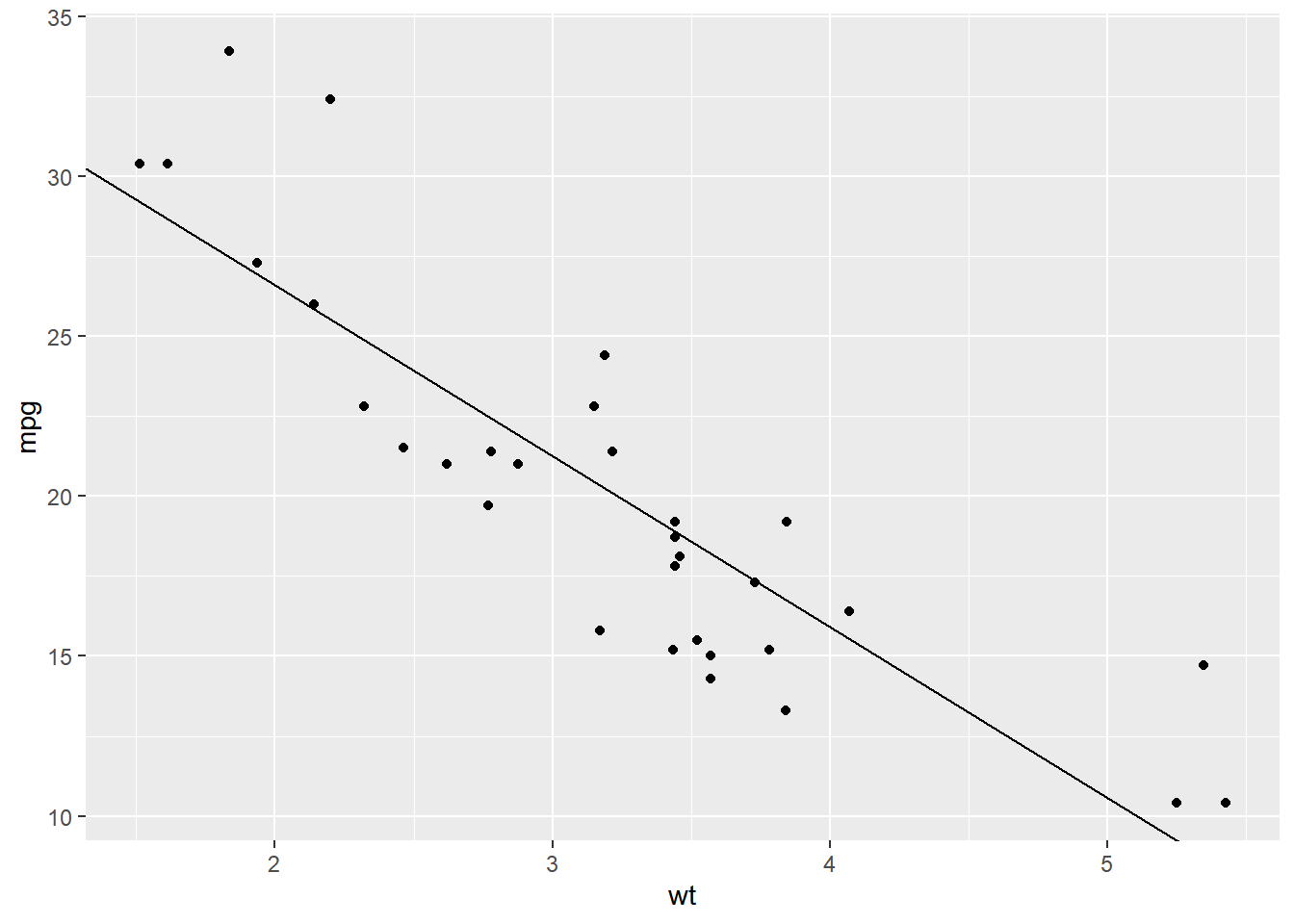#### Regression Line

If you are using `geom_smooth()`, you need to specify the method of fitting the line, which can be `lm` or `loess`. You also need to indicate whether the confidence interval must be displayed using the `se` argument.

``````ggplot(mtcars, aes(x = wt, y = mpg)) +
geom_smooth(method = 'lm', se = TRUE)``````
``## `geom_smooth()` using formula 'y ~ x'``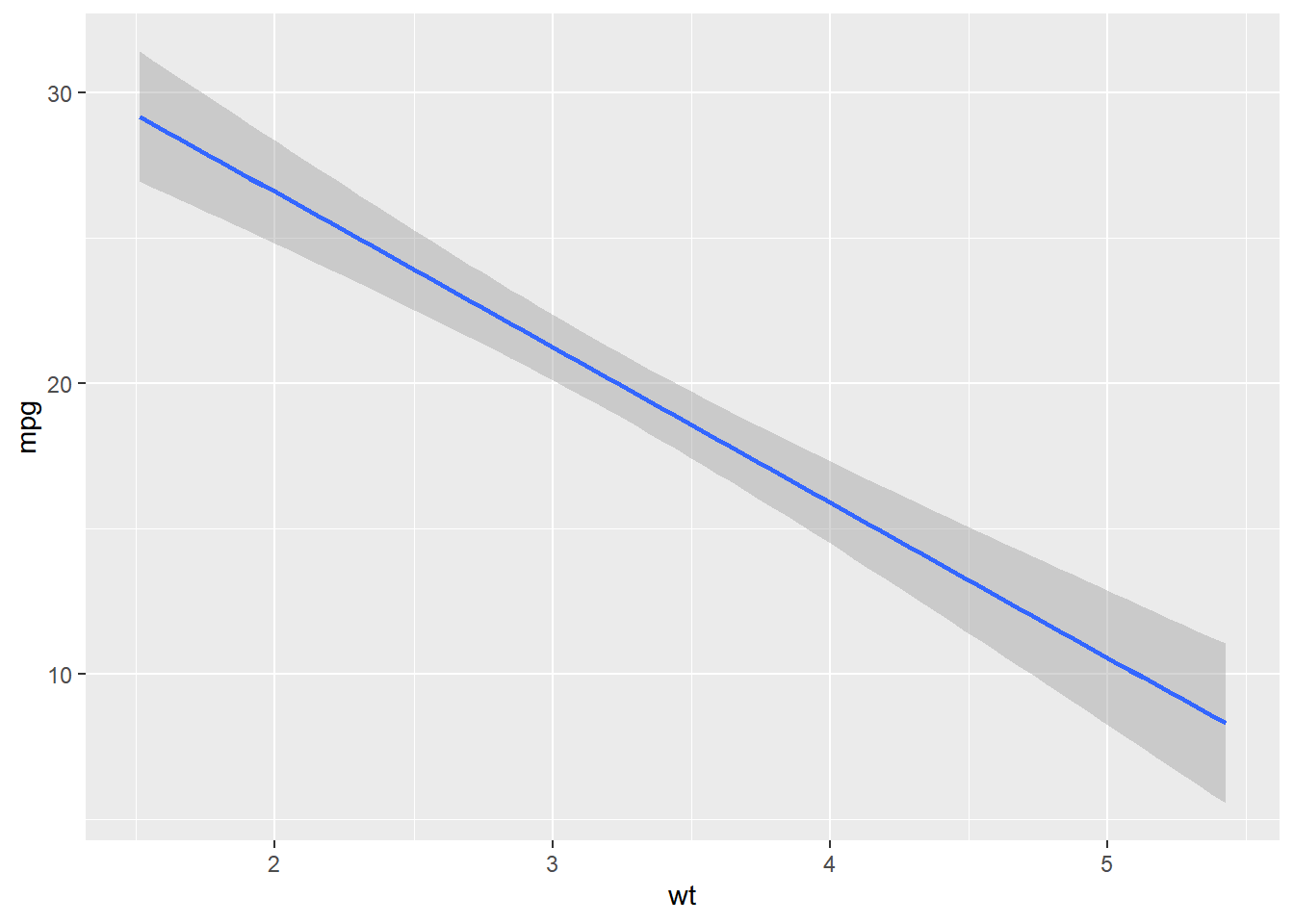#### Loess Method

Here we use the `'loess'` method to fit the regression line.

``````ggplot(mtcars, aes(x = wt, y = mpg)) +
geom_smooth(method = 'loess', se = FALSE)``````
``## `geom_smooth()` using formula 'y ~ x'``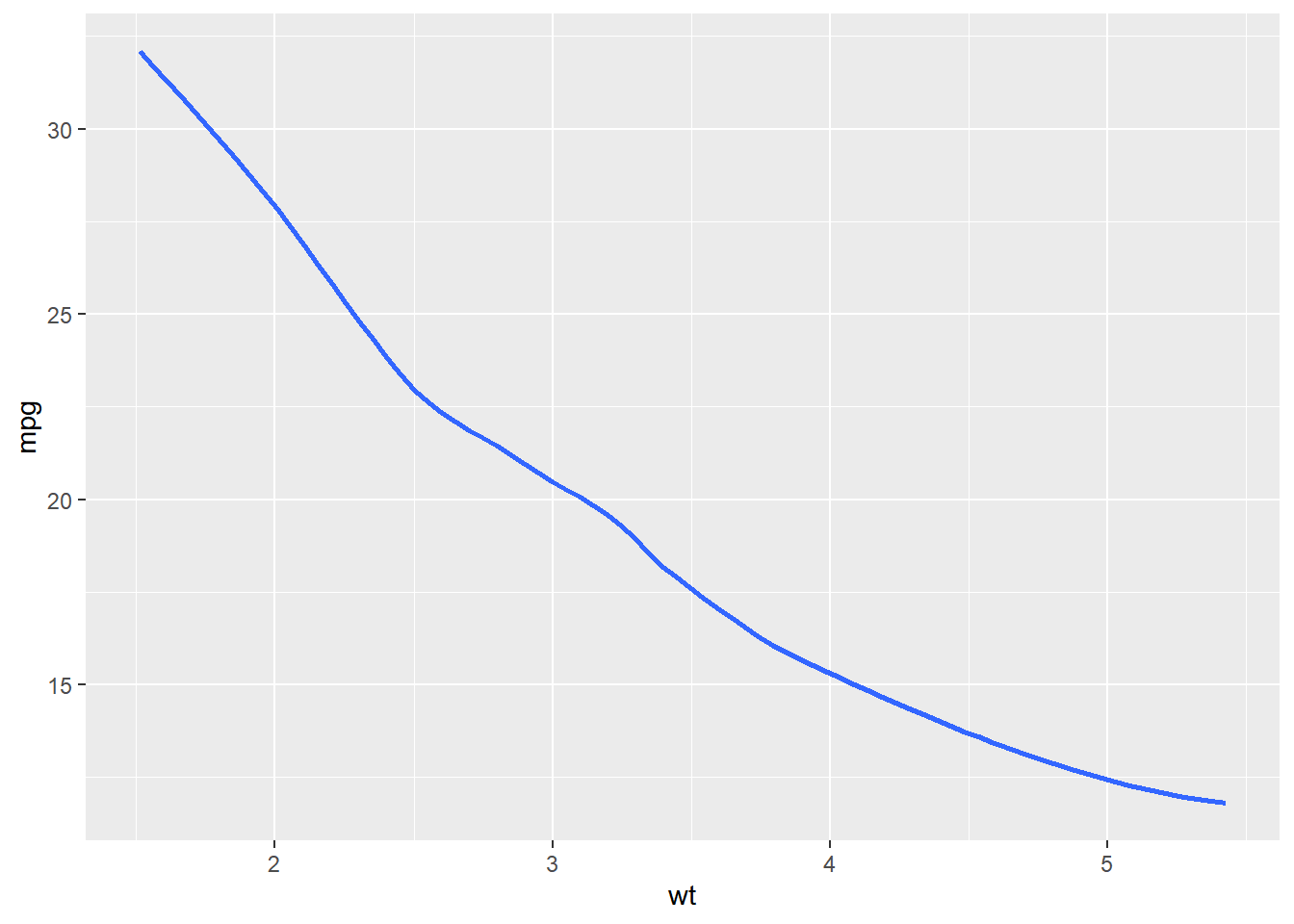#### Horizontal/Vertical Lines

Add horizontal or vertical lines using

• `geom_hline()`
• `geom_vline()`

#### Horizontal Line

To add a horizontal line, the Y axis intercept must be supplied using the `yintercept` argument.

``````ggplot(mtcars, aes(x = wt, y = mpg)) +
geom_point() +
geom_hline(yintercept = 30) ``````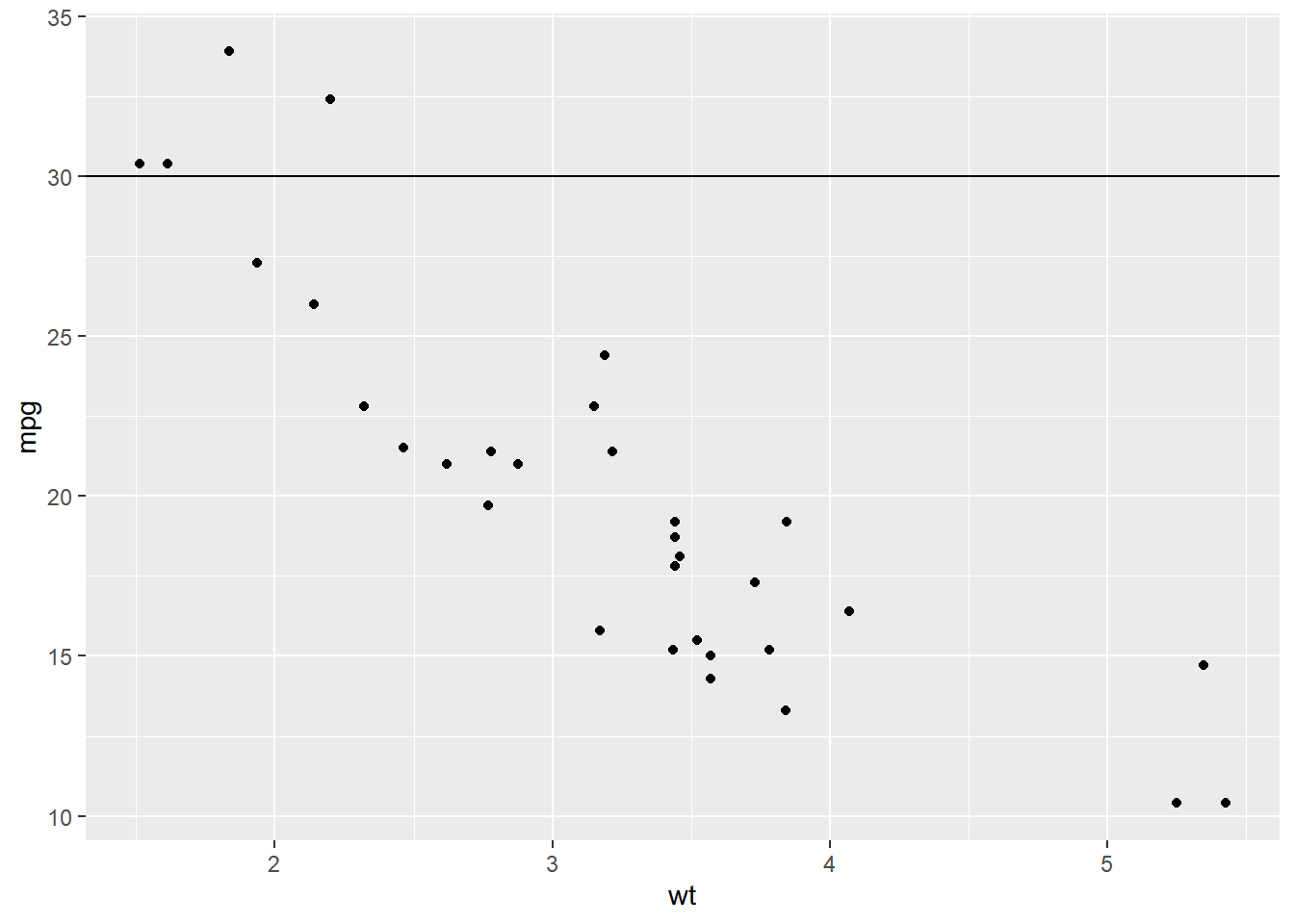#### Vertical Line

For the vertical line, the X axis intercept must be supplied using the `xintercept` argument.

``````ggplot(mtcars, aes(x = wt, y = mpg)) +
geom_point() +
geom_vline(xintercept = 5) ``````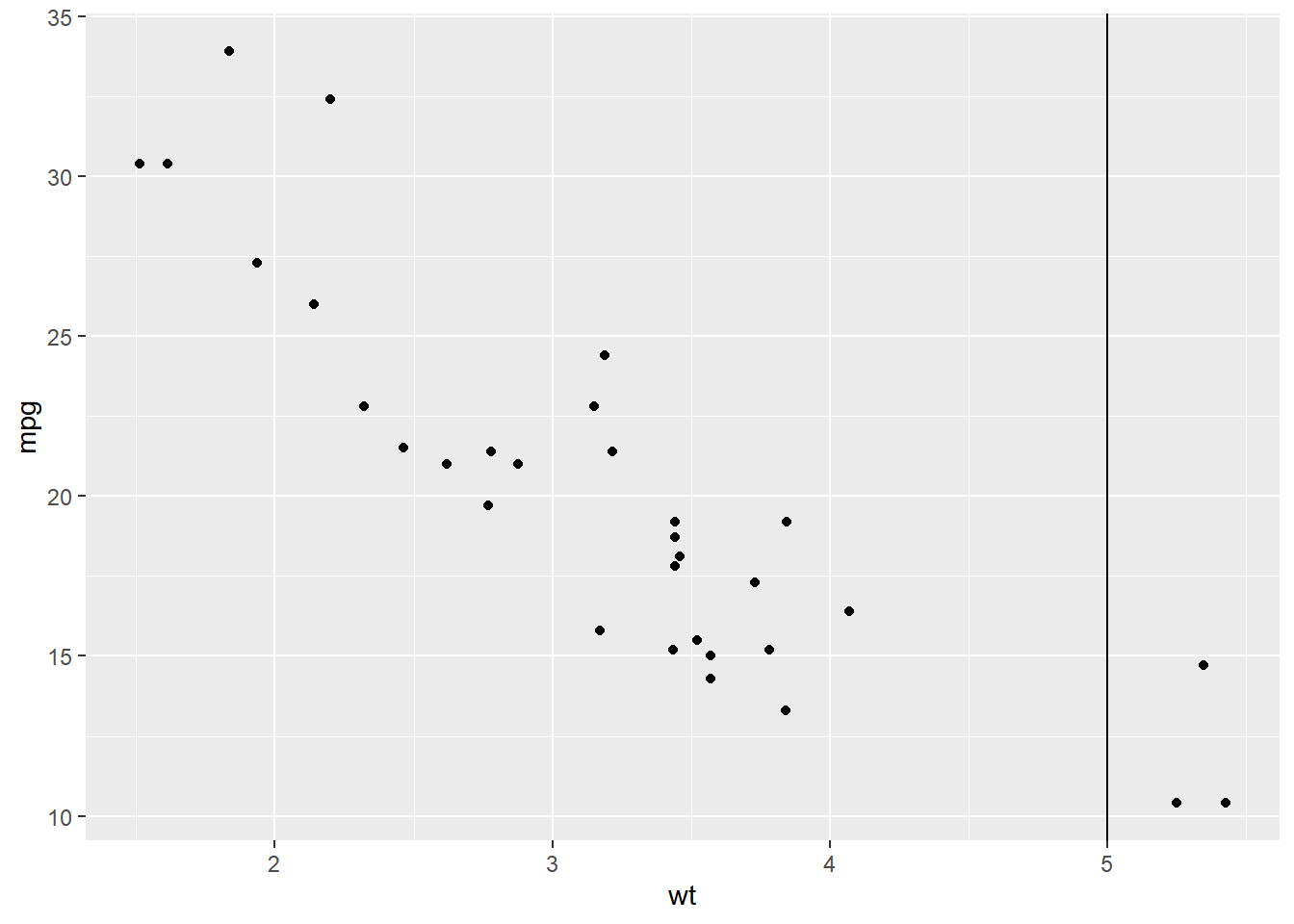#### Bar Plot

Bar plots present grouped data with rectangular bars. The bars may represent the frequency of the groups or values. Bar plots can be:

• horizontal
• vertical
• grouped
• stacked
• proportional

#### Frequency

``````ggplot(ecom, aes(x = factor(device))) +
geom_bar()``````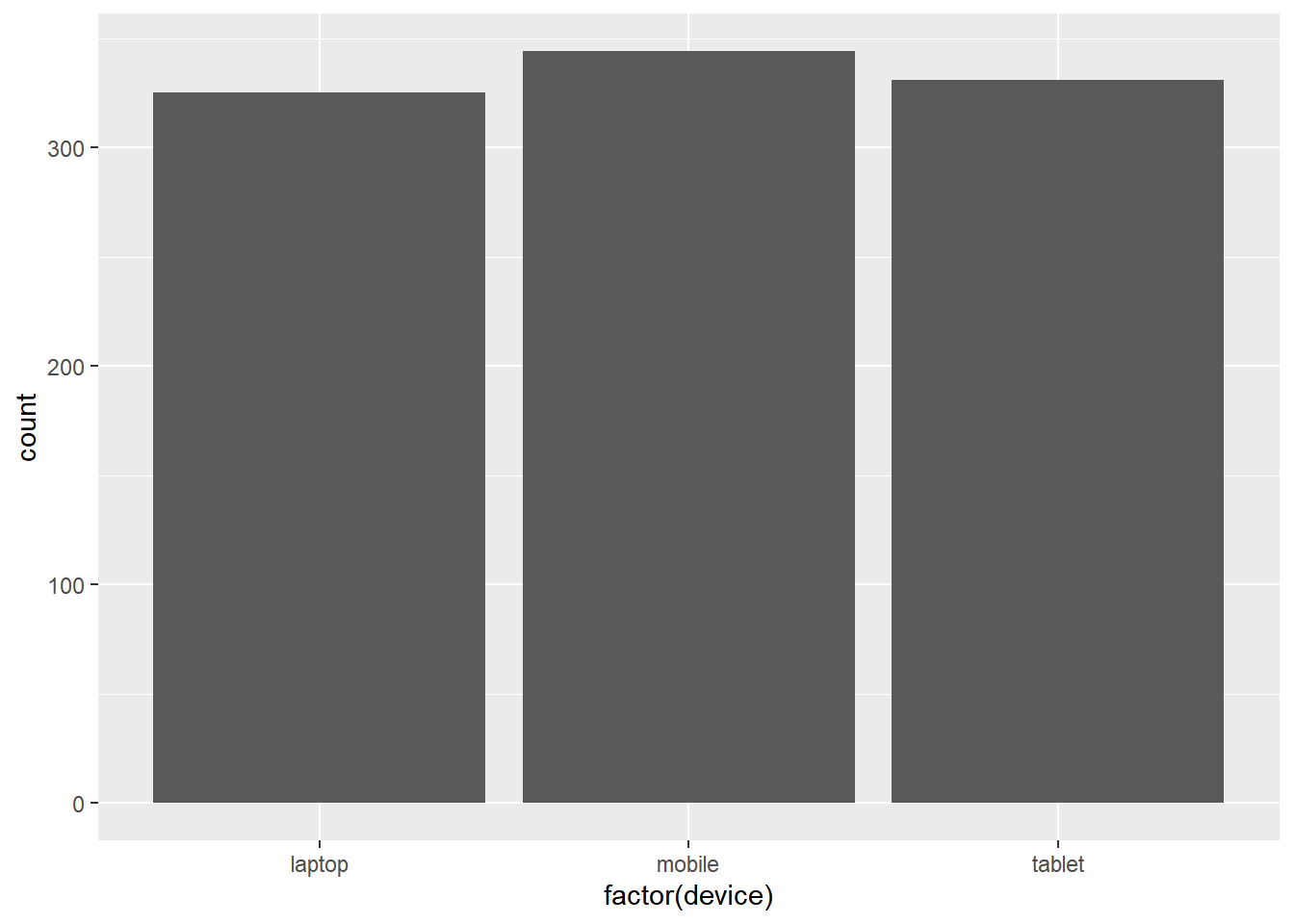#### Weight

If the bars should represent a continuous variable, use the `weight` argument within `aes()`. In the below example, the bars do not represent the count of devices, instead, they represent the total order value for each device type.

``````ggplot(ecom, aes(x = factor(device))) +
geom_bar(aes(weight = order_value))``````#### Stacked Bar Plot

To create a stacked bar plot, the `fill` argument must be mapped to a categorical variable.

``````ggplot(ecom, aes(x = factor(device))) +
geom_bar(aes(fill = purchase))``````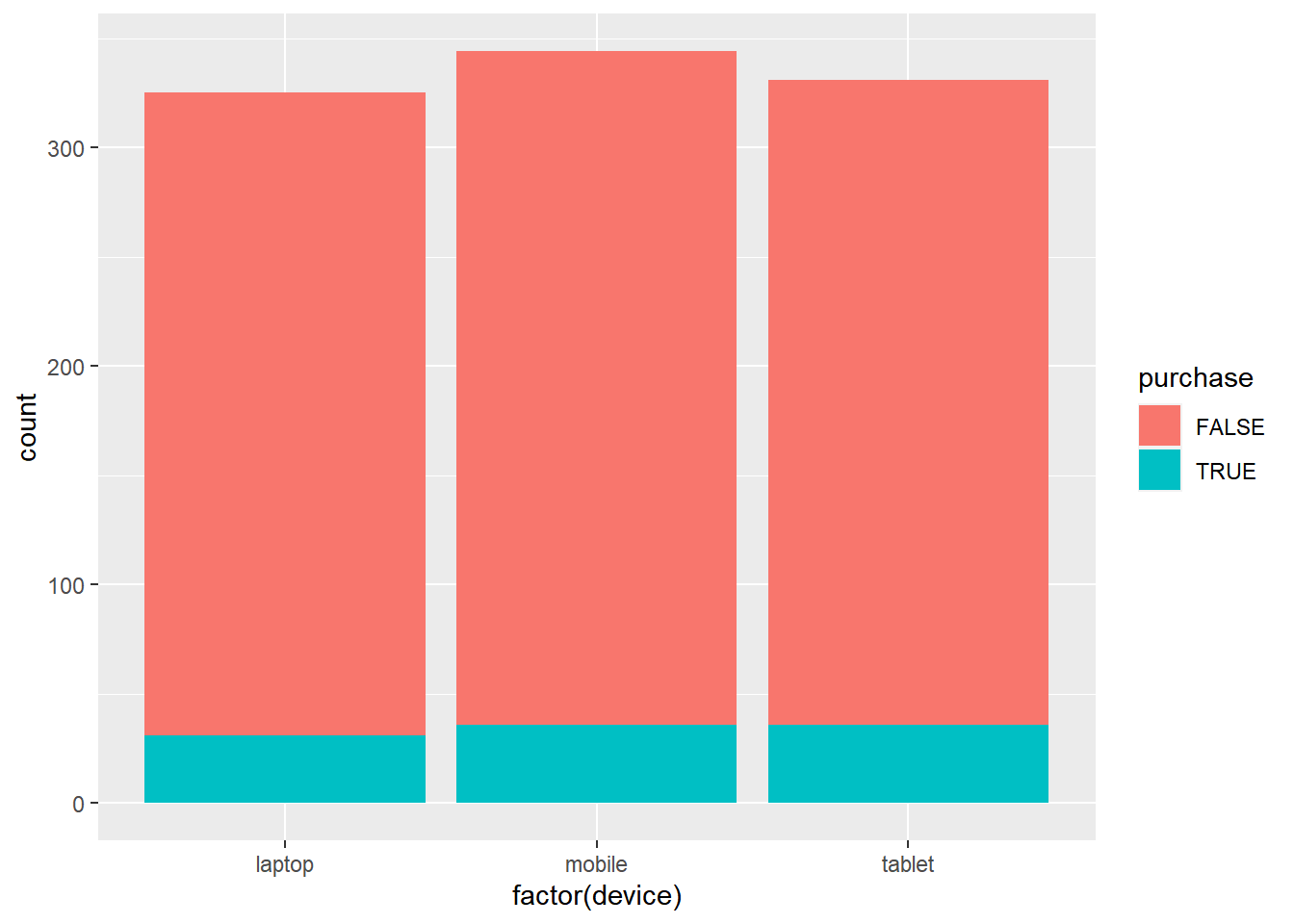#### Horizontal Bar Plot

A horizontal bar plot can be created by flipping the coordinate axes using the `coord_flip()` function.

``````ggplot(ecom, aes(x = factor(device))) +
geom_bar(aes(fill = purchase)) +
coord_flip()``````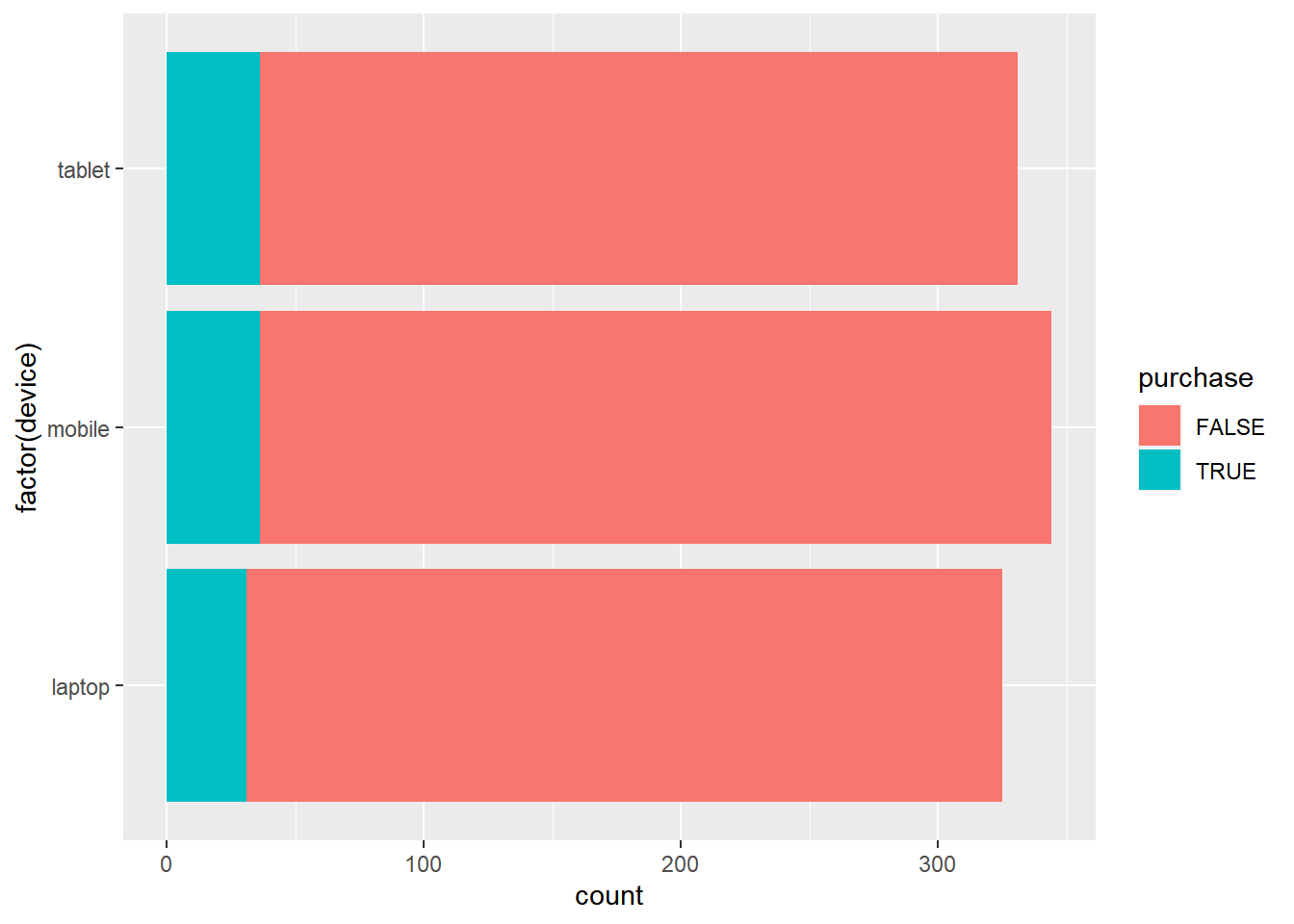#### Columns

If the data has already been summarized, you can use `geom_col()` instead of `geom_bar()`. In the below example, we have the total visits for each device type. The data has already been summarized and as such we cannot use `geom_bar()`.

``````device <- c('laptop', 'mobile', 'tablet')
visits <- c(30000, 12000, 5000)
traffic <- tibble::tibble(device, visits)
ggplot(traffic, aes(x = device, y = visits)) +
geom_col(fill = 'blue') ``````#### Boxplot

The box plot is a standardized way of displaying the distribution of data based on the five number summary: minimum, first quartile, median, third quartile, and maximum. Box plots are useful for detecting outliers and for comparing distributions. It shows the shape, central tendancy and variability of the data. Use `geom_boxplot()` to create a box plot.

``````ggplot(ecom, aes(x = factor(device), y = n_pages)) +
geom_boxplot()``````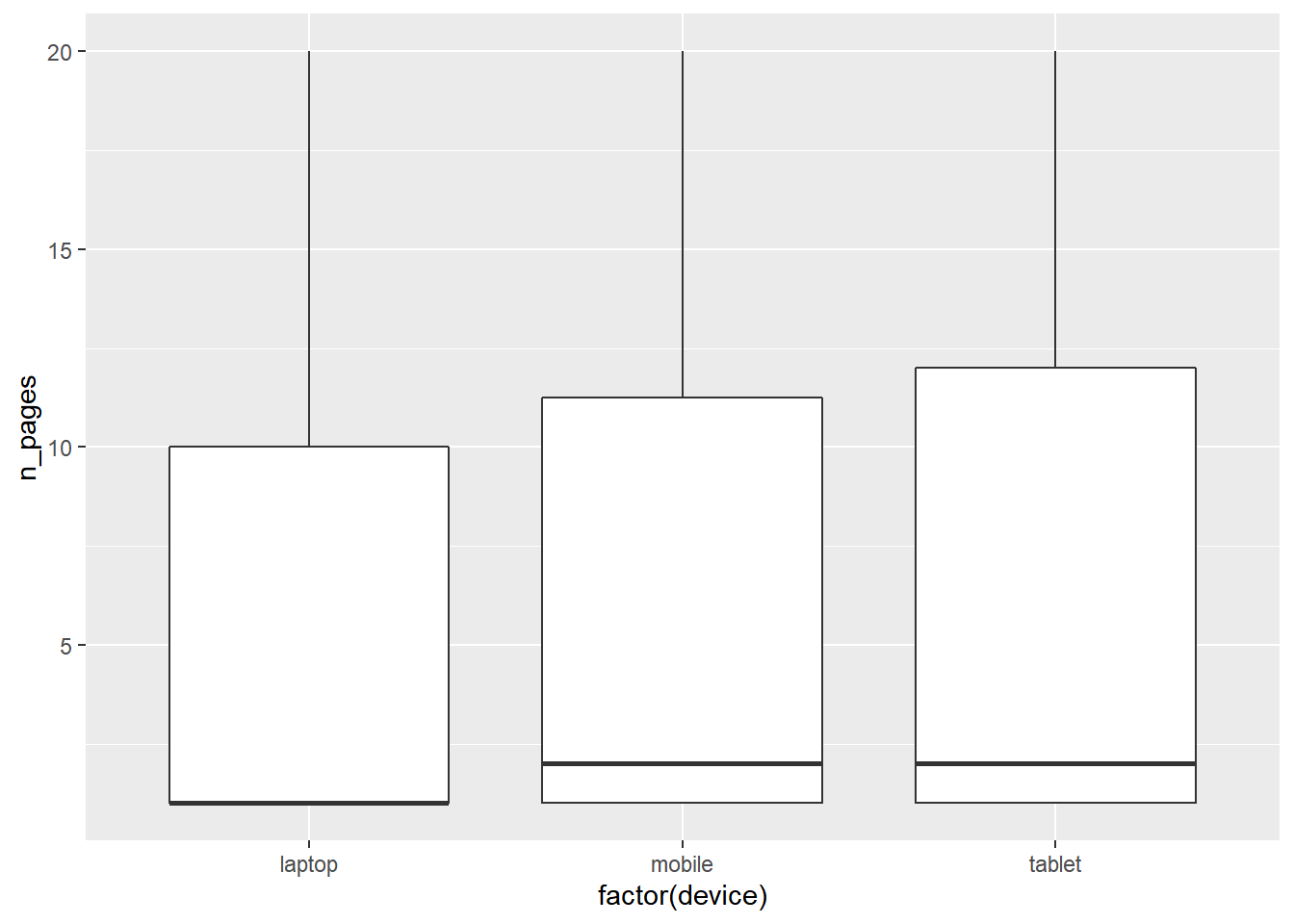#### Histogram

A histogram is a plot that can be used to examine the shape and spread of continuous data. It looks very similar to a bar graph and can be used to detect outliers and skewness in data. Use `geom_histogram()` to create a histogram.

``````ggplot(ecom, aes(x = duration)) +
geom_histogram()``````
``## `stat_bin()` using `bins = 30`. Pick better value with `binwidth`.``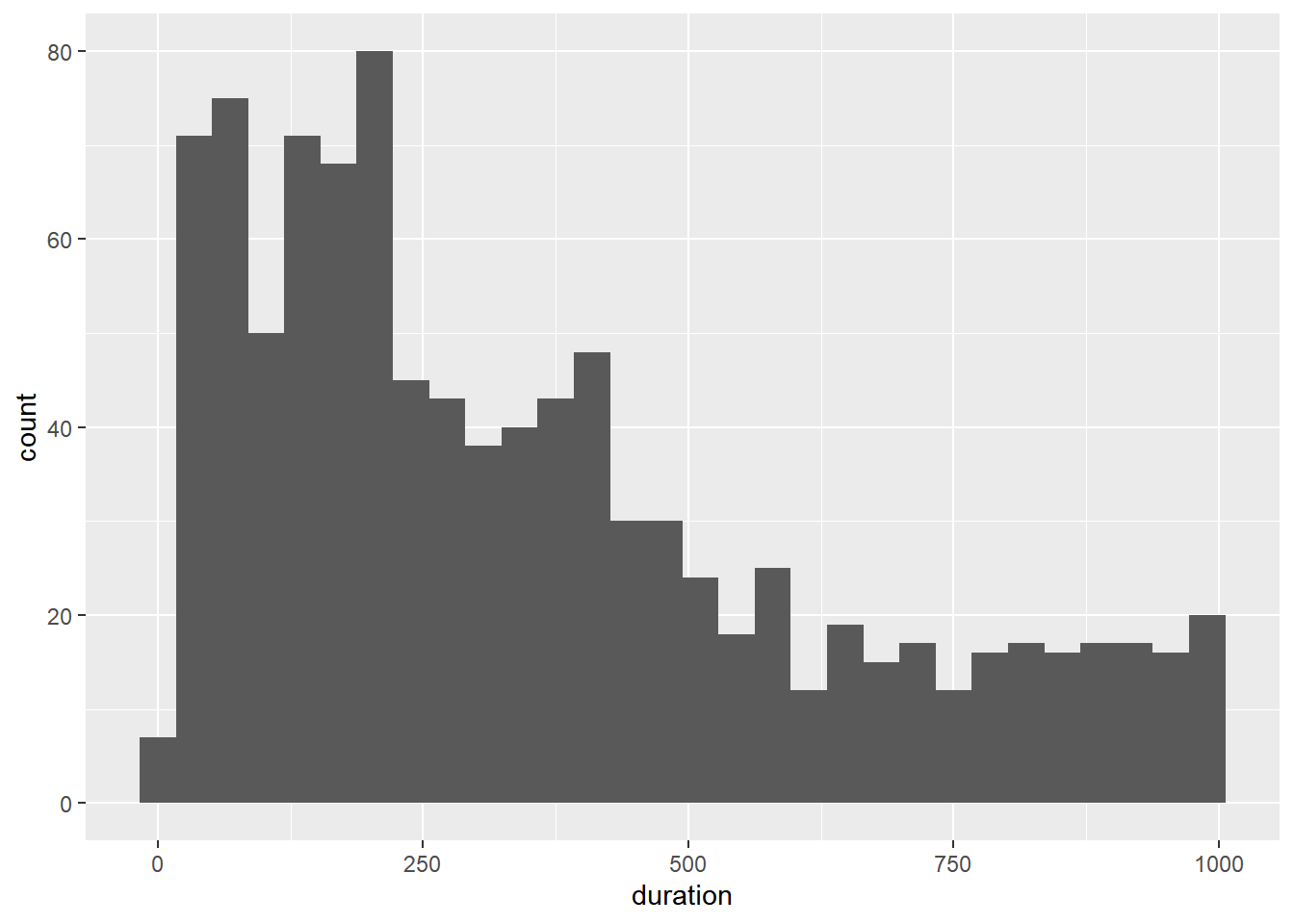You can control the number of bins using the `bins` argument.

``````ggplot(ecom, aes(x = duration)) +
geom_histogram(bins = 5)``````#### Line

Line charts are used to examine trends over time. We will use a different data set for exploring line plots.

#### Data

``gdp <- readr::read_csv('https://raw.githubusercontent.com/rsquaredacademy/datasets/master/gdp.csv')``
``## Warning: Missing column names filled in: 'X1' ``
``gdp``
``````## # A tibble: 6 x 6
##      X1     X year       growth india china
##   <dbl> <dbl> <date>      <dbl> <dbl> <dbl>
## 1     1     1 2000-01-01      6     5     8
## 2     2     2 2001-01-01      9     9     5
## 3     3     3 2002-01-01      8     8     6
## 4     4     4 2003-01-01      9     8     8
## 5     5     5 2004-01-01      9     5     9
## 6     6     6 2005-01-01      8     7     8``````

Use `geom_line()` to create a line chart. In the below plot, we chart the GDP of India, the fastest growing economy in emerging markets, across years.

``````ggplot(gdp, aes(year, india)) +
geom_line()``````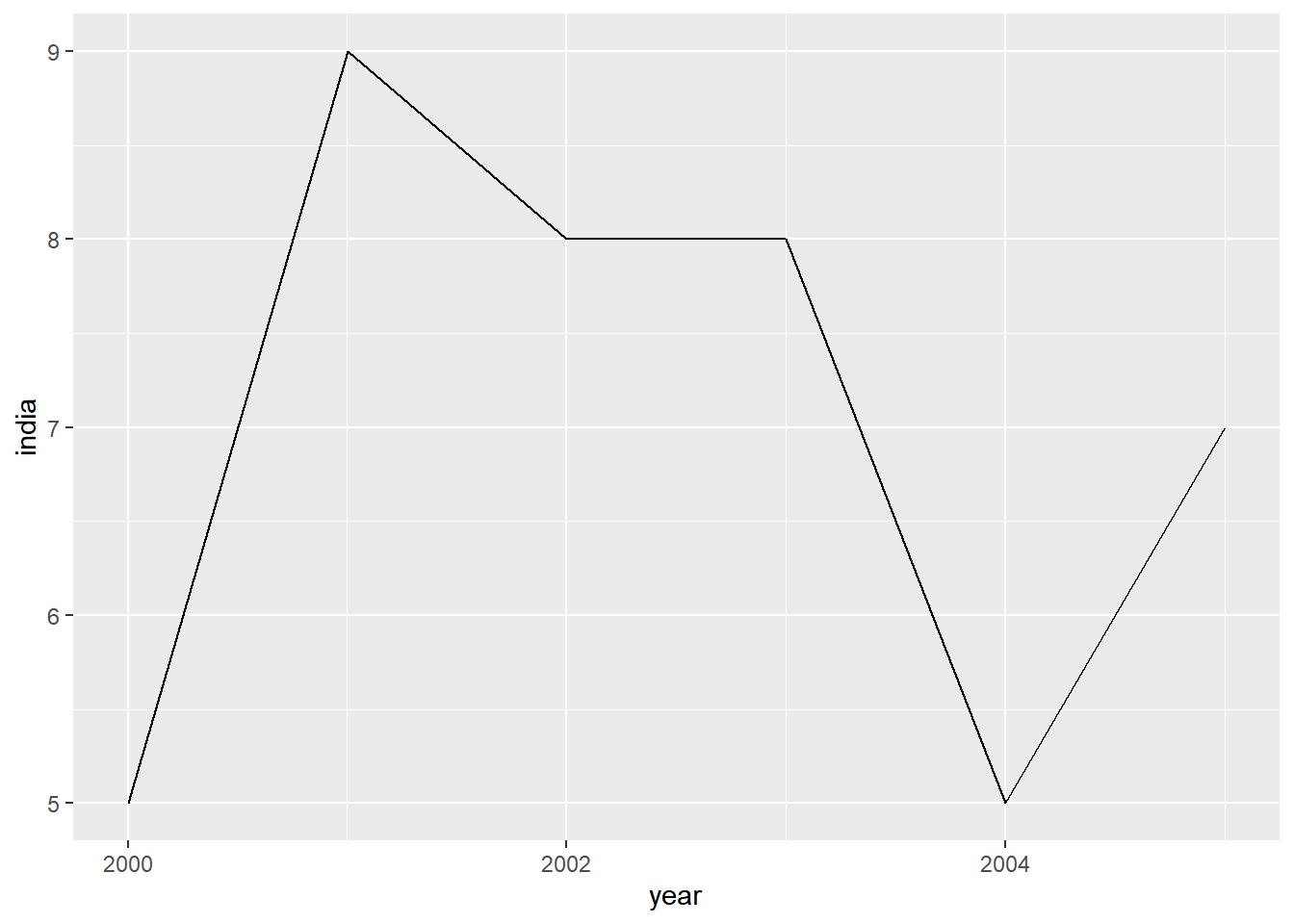The color and line type can be modified using the `color` and `linetype` arguments. We will explore the different line types in an upcoming post.

``````ggplot(gdp, aes(year, india)) +
geom_line(color = 'blue', linetype = 'dashed')``````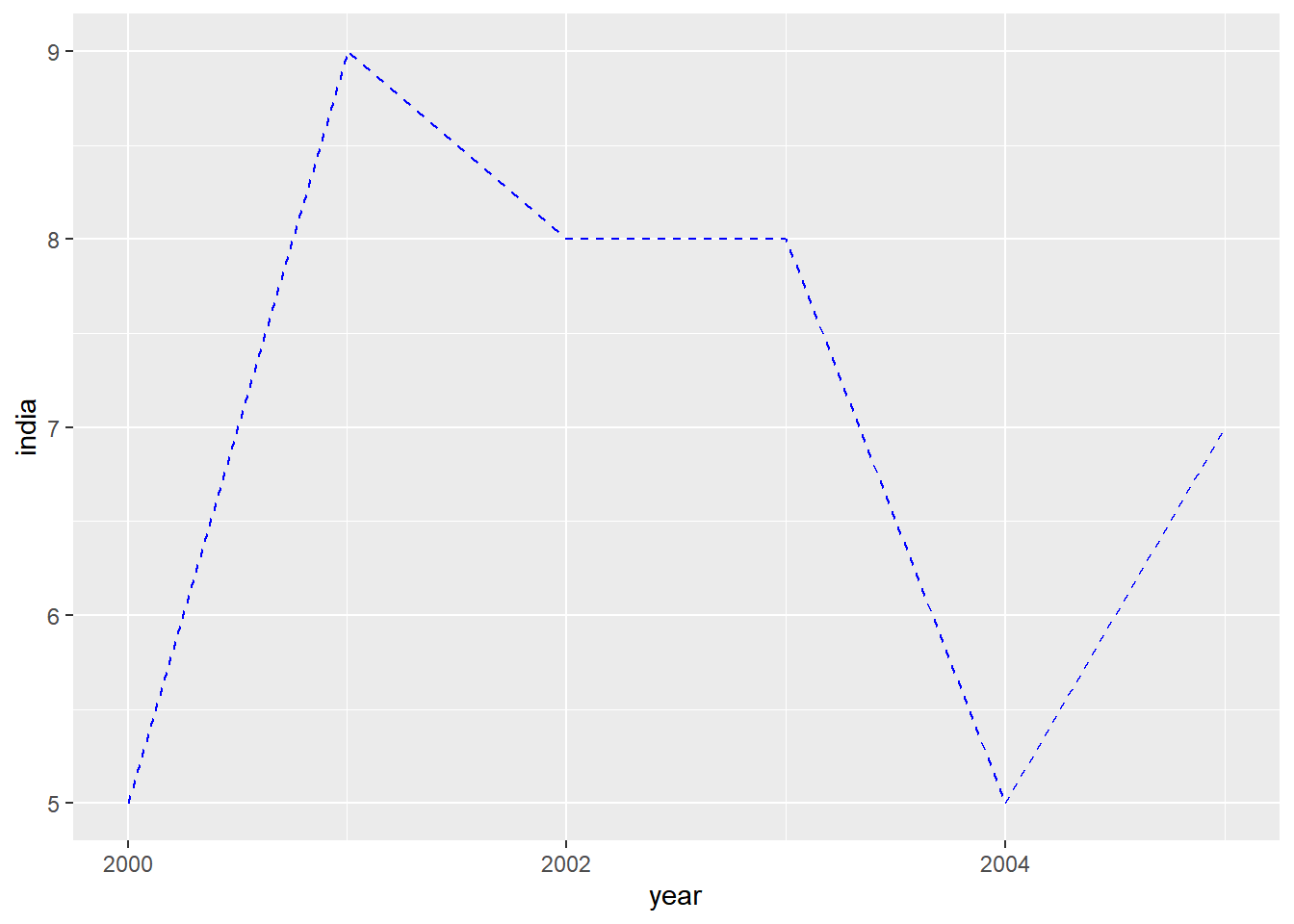#### Label

You can label the points using `geom_label()`.

``````ggplot(mtcars, aes(disp, mpg, label = rownames(mtcars))) +
geom_label()``````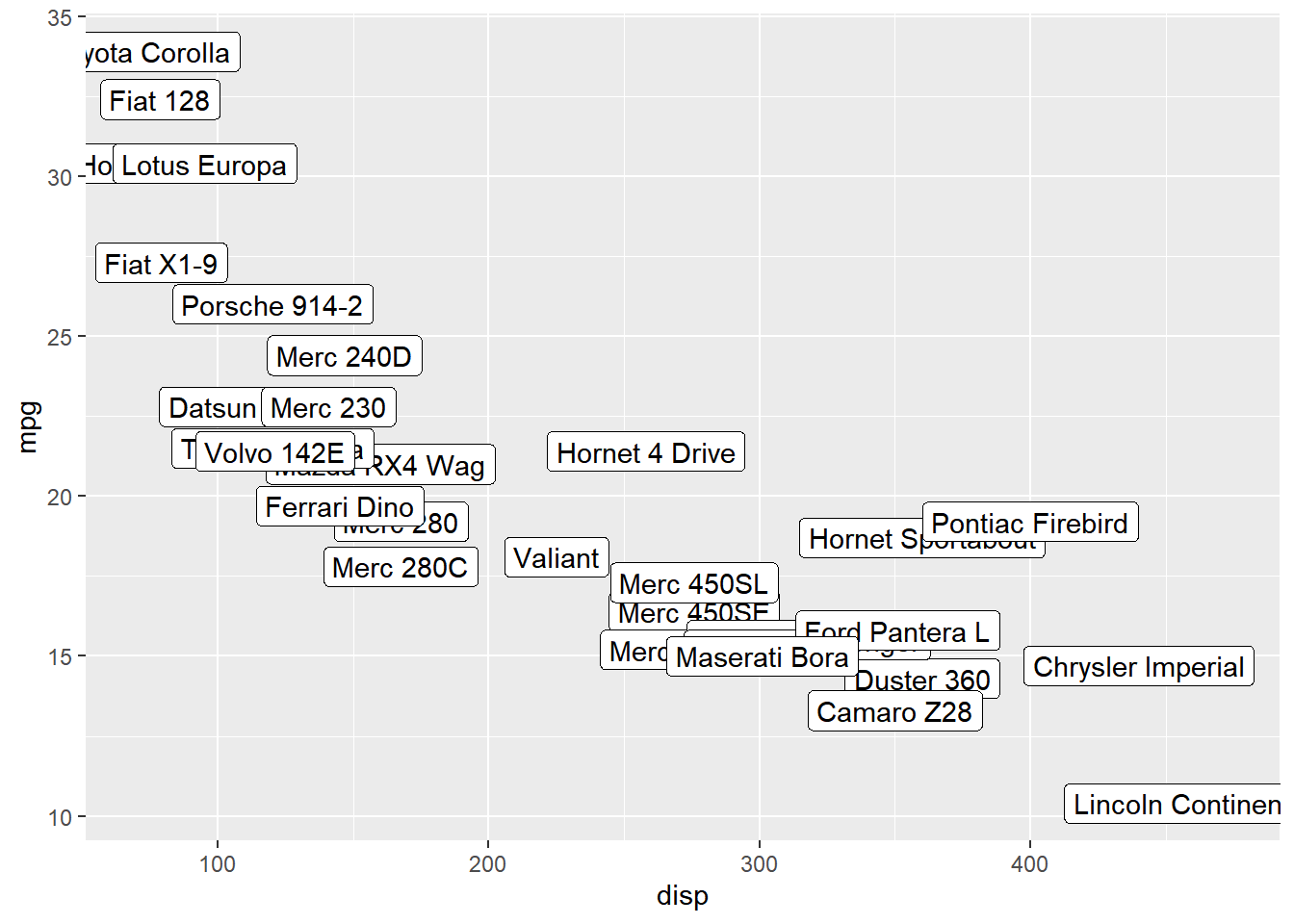#### Text

`geom_text()` offers another way to add text to the plots. We will learn to modify the appearance and location of the text in another post.

``````ggplot(mtcars, aes(disp, mpg, label = rownames(mtcars))) +
geom_text(check_overlap = TRUE, size = 2)``````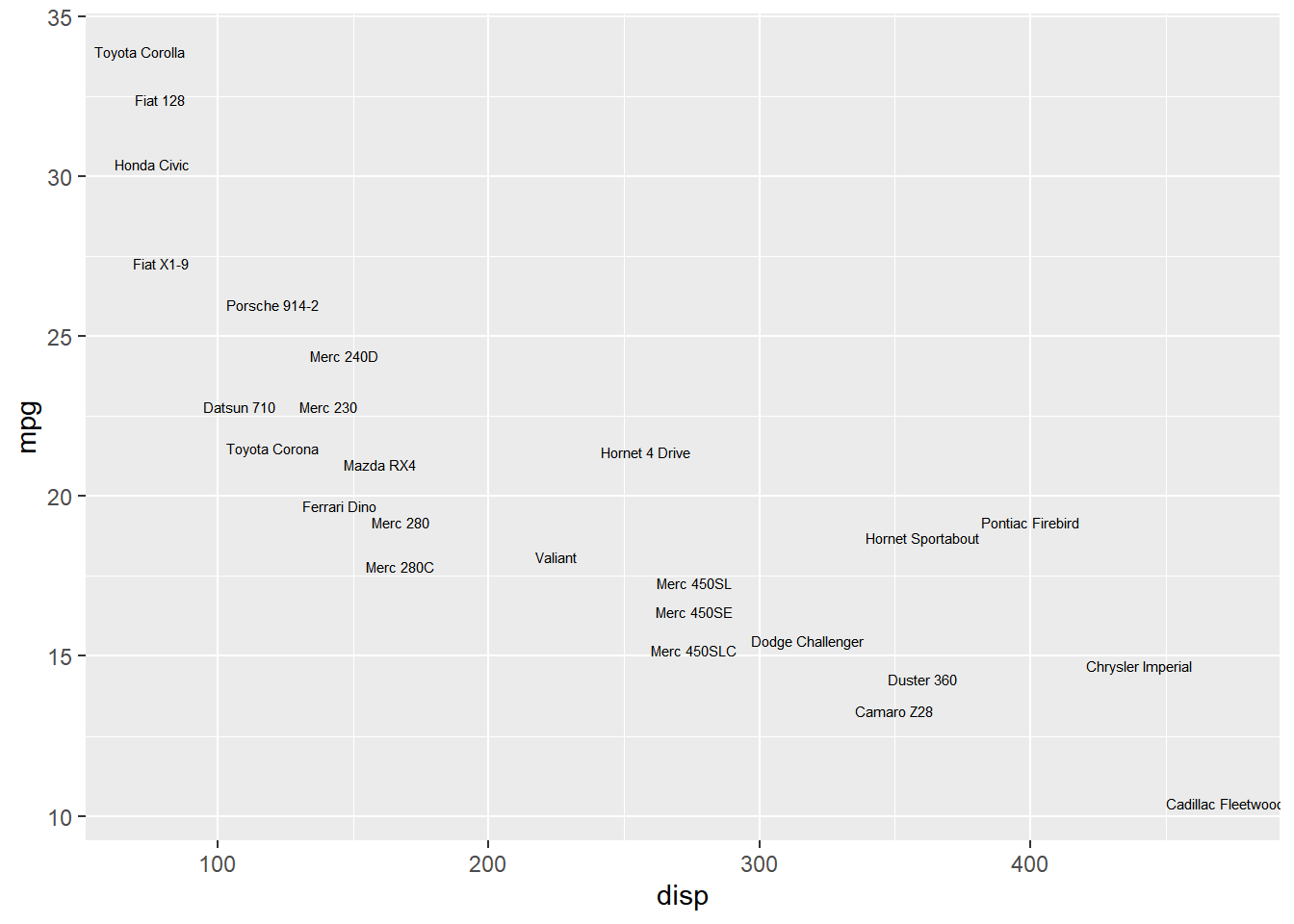### Summary

In this post, we learnt about different `geoms` such as

• `geom_point()`
• `geom_line()`
• `geom_histogram()`
• `geom_bar()`
• `geom_boxplot()`
• `geom_abline()`
• `geom_text()`

### Up Next..

In the next post, we will learn about aesthetics.